Isaac's Blog

Tutte Embedding for Parameterization

2018/03/19In terms of geometry processing, Tutte embedding, also known as barycentric embedding, can be used for mesh parameterization by fixing the boundary vertices of the mesh on a certain convex polygon, and building a crossing-free straight-line embedding with the interior vertices inside the convex boundary.

Implementation of Tutte Embedding

In order to implement a Tutte embedding, we have to use two vectors $u$ and $v$ to store the parameterized coordinates, and another two vectors $\bar u$ and $\bar v$ to store the boundary vertices. For the interior vertex $i$, we can directly applying the following equation on it to build the barycentric mapping, $j$ denotes the adjacent vertices of $i$.

$$\begin{equation} \sum_{}a_{i,j} \begin{pmatrix} u_j \\ v_j \end{pmatrix} -a_{i,i} \begin{pmatrix} u_i \\ v_i \end{pmatrix} =0 \end{equation}$$

For those vertices on the boundary, we use the equation below and set $a_{i,i}=1$, to have them fixed on a convex shape:

$$\begin{equation} \sum_{}a_{i,i} \begin{pmatrix} u_i \\ v_i \end{pmatrix} = \begin{pmatrix} \bar u_i \\ \bar v_i \end{pmatrix} \end{equation}$$

Thus, we can build the two linear systems in below, where $A=\{a_{i,j}\}$ is a sparse matrix storing all the weights $a_{i,j}$, and find the parameterized coordinates by solving for them.

$$\begin{equation} \begin{cases} Au=\bar u \\ Av=\bar v \end{cases} \end{equation}$$

I chose a disk as the convex shape for fixing the boundary vertices. And I am still using geometry-processing-js as mesh library, which is the same library I used in Mesh Smoothing.

Weighting Schemes

I tried three different weight sets, namely the uniform Laplacian weights, the Laplace-Beltrami weights, and the mean value weights.

Uniform Laplacian Weights

The uniform Laplacian weight set is easiest to build, but it does not take the geometric feature of the mesh into consideration. We can simply set the entries of the weight matrix in the following manner:

$$\begin{equation} \begin{cases} a_{i,j}=1 \\ a_{i,i}=-\sum_{}a_{i,j}=-degree_i \end{cases} \end{equation}$$

Laplace-Beltrami Weights

We can also derive weights from the Laplace-Beltrami operator:

$$\begin{equation} \begin{cases} a_{i,j}=\frac{1}{2A_i}(\cot\alpha_{i,j}+\cot\beta_{i,j}) \\ a_{i,i}=-\sum_{}a_{i,j} \end{cases} \end{equation}$$

In the equations above, $A_i$ denotes the area of the Voronoi region of the vertex $i$, $\alpha_{i,j}$ and $\beta_{i,j}$ denote respectively the two corners opposite to the edge $(i,j)$, as is shown in Figure 1.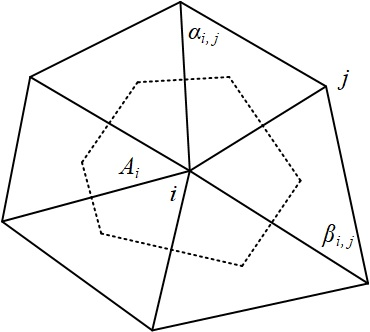Figure 1: Voronoi region of one vertex.

For calculating the value of $A_i$, we can use this formula, where $\Arrowvert x_i-x_j \Arrowvert$ is the length of edge $(i,j)$.

$$\begin{equation} A_i=\frac{1}{8}\sum_{}(\cot\alpha_{i,j}+\cot\beta_{i,j})\Arrowvert x_i-x_j \Arrowvert^2 \end{equation}$$

As far as one triangle from the one-ring of a vertex is concerned, which is shown in Figure 2, the Voronoi region inside that triangle can be calculated as:

$$\begin{equation} W=\frac{1}{8}\sum_{}(\Arrowvert x_i-x_j \Arrowvert^2\cot\alpha+\Arrowvert x_i-x_j \Arrowvert^2\cot\beta) \end{equation}$$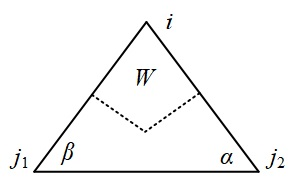Figure 2: Voronoi region of one triangle of one-ring.

As the data structure of mesh is based on half-edge, we can simply find the neighbor of a certain half-edge by retrieving its previous half-edge, e.g., in Figure 2, half-edge $(j_2,i)$ is previous to half-edge $(i,j_1)$. Thus, by traversing outgoing half-edges associated on a vertex, we can easily calculate the Voronoi region of it using the following code snippet:

Mean Value Weights

The Laplace-Beltrami weight takes the geometry of a mesh into account, but it could be negative with obtuse triangles. Based on the mean value theorem, we can the mean value weights, which are always positive and generate one-to-one mappings.

$$\begin{equation} \begin{cases} a_{i,j}=\frac{1}{\Arrowvert x_i-x_j \Arrowvert}(\tan(\frac{\delta_{i,j}}{2})+\tan(\frac{\gamma_{i,j}}{2})) \\ a_{i,i}=-\sum_{}a_{i,j} \end{cases} \end{equation}$$

In the equations above, $\Arrowvert x_i-x_j \Arrowvert$ is the length of edge $(i,j)$, $\delta_{i,j}$ and $\gamma_{i,j}$ are two neighboring corners on both sides of edge $(i,j)$, as is shown in Figure 3.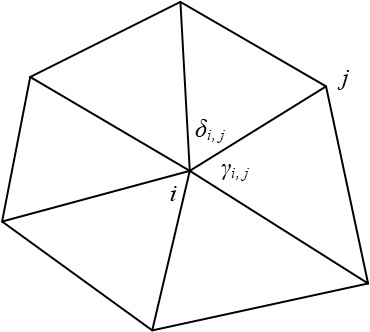Figure 3

In the real implementation, like what we did in calculating the Voronoi region, we can still take advantage of the sequence of half-edges to find the corresponding corners:

Results and Conclusions

The results of Tutte embedding is shown in Figure 4, applied on different objects with different weight sets.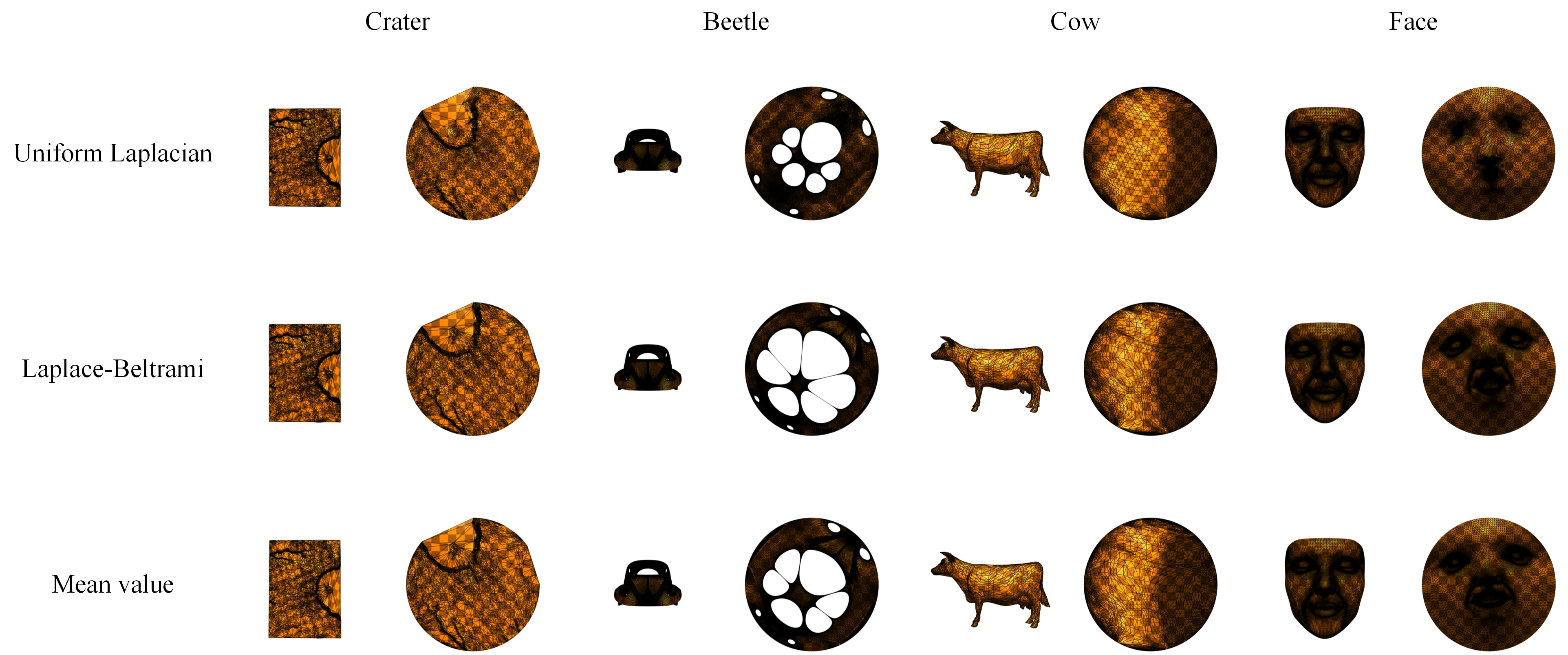Figure 4: Results of Tutte embedding.

We can conclude from the results that the Laplace-Beltrami weight set and mean value weight set can better preserve the shape of triangles in the original mesh. This leads to the interior holes of a mesh to enlarge, which is clearly shown in beetle. For better illustrating this property, we can take a close look at the mesh (Figure 5).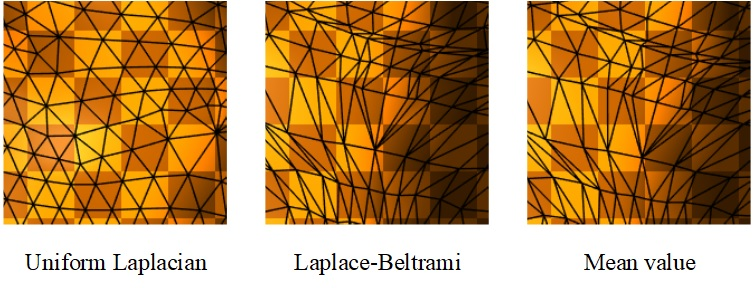Figure 5: Parts of parameterizations of cow.

Figure 5 takes the same 200×200 pixels parts from the screen shots of the parametrizations of cow object. It shows that uniform Laplacian weight can cause the triangles on the parameterization to become more regular, but the triangles on the parameterized mesh of Laplace-Beltrami weight and mean value weight are more similar to those on the original mesh.

Besides, Laplace-Beltrami weight and mean value weight can even better preserve the patterns on the mesh. As is shown in Figure 6, the features on the face object (i.e. mouth and eyes) are more distinguishable if we use Laplace-Beltrami weight or mean value weight.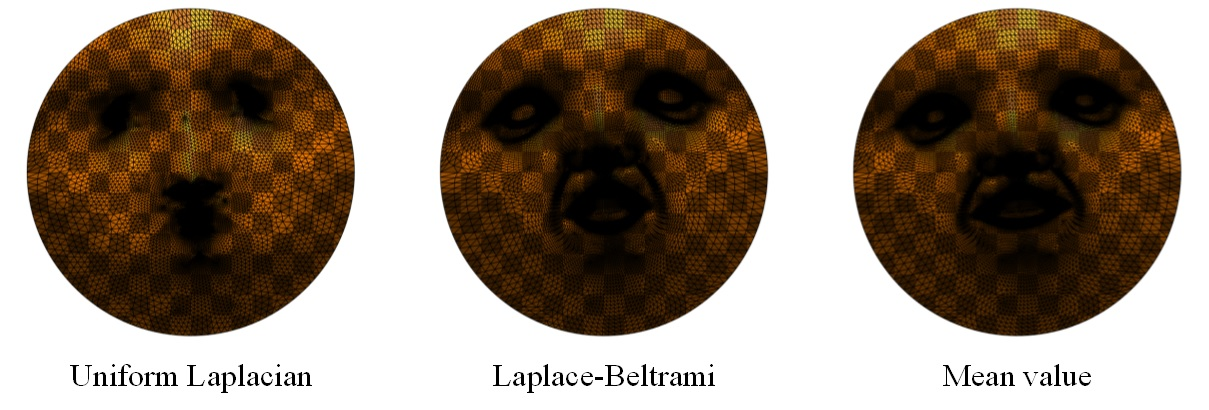Figure 6: Parameterizations of face.

I have also uploaded the project to this blog: /projects/tutte-embedding, we can go there to see how the algorithms work.